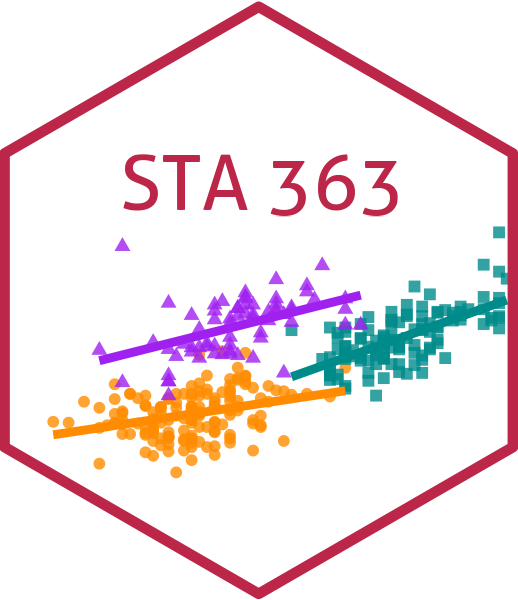# Bagging Decision Trees

Lucy D’Agostino McGowan

## Decision trees

### Pros

• simple
• easy to interpret

### Cons

• not often competitive in terms of predictive accuracy
• we will discuss how to combine multiple trees to improve accuracy
• Ensemble methods

## Bagging

• bagging is a general-purpose procedure for reducing the variance of a statistical learning method (outside of just trees)

• It is particularly useful and frequently used in the context of decision trees

• Also called bootstrap aggregation

## Bagging

• Mathematically, why does this work? Let’s go back to intro to stat!

• If you have a set of $n$ independent observations: $Z_1, \dots, Z_n$, each with a variance of $\sigma^2$, what would the variance of the mean, $\bar{Z}$ be?

• The variance of $\bar{Z}$ is $\sigma^2/n$

• In other words, averaging a set of observations reduces the variance.

• This is generally not practical because we generally do not have multiple training sets

## Bagging

Averaging a set of observations reduces the variance. This is generally not practical because we generally do not have multiple training sets.

What can we do?

• Bootstrap! We can take repeated samples from the single training data set.

## Bagging process

• generate $B$ different bootstrapped training sets

• Train our method on the $b$th bootstrapped training set to get $\hat{f}^{*b}(x)$, the prediction at point $x$

• Average all predictions to get: $\hat{f}_{bag}(x)=\frac{1}{B}\sum_{b=1}^B\hat{f}^{*b}(x)$

• This is bagging!

## Bagging regression trees

• generate $B$ different bootstrapped training sets
• Fit a regression tree on the $b$th bootstrapped training set to get $\hat{f}^{*b}(x)$, the prediction at point $x$
• Average all predictions to get: $\hat{f}_{bag}(x)=\frac{1}{B}\sum_{b=1}^B\hat{f}^{*b}(x)$

## Bagging classification trees

• for each test observation, record the class predicted by the $B$ trees

• Take a majority vote - the overall prediction is the most commonly occuring class among the $B$ predictions

## Out-of-bag Error Estimation

• You can estimate the test error of a bagged model

• The key to bagging is that trees are repeatedly fit to bootstrapped subsets of the observations

• On average, each bagged tree makes use of about 2/3 of the observations (you can prove this if you’d like!, not required for this course though)

• The remaining 1/3 of observations not used to fit a given bagged tree are the out-of-bag (OOB) observations

## Out-of-bag Error Estimation

You can predict the response for the $i$th observation using each of the trees in which that observation was OOB

How many predictions do you think this will yield for the $i$th observation?

• This will yield $B/3$ predictions for the $i$th observations. We can average this!

• This estimate is essentially the LOOCV error for bagging as long as $B$ is large 🎉

## Describing Bagging

See if you can draw a diagram to describe the bagging process to someone who has never heard of this before.

05:00